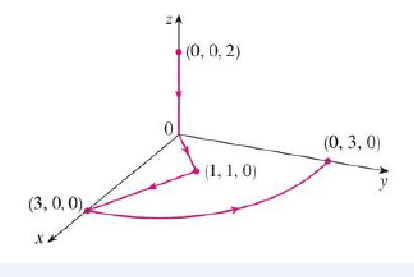Chapter 16, Problem 37RE

Chapter
Section
Textbook Problem

Let F(x, y, z) = (3x2 yz – 3y) i + (x3z – 3x) j + (x3y + 2z) kEvaluate ∫C F • dr, where C is the curve with initial point (0, 0, 2) and terminal point (0, 3, 0) shown in the figure.To determine

To evaluate: The value of CFdr , where C is the curve with initial point (0,0,2) and terminal point (0,3,0) .

Explanation

Given data:

F(x,y,z)=(3x2yz3y)i+(x3z3x)j+(x3y+2z)k (1)

Formula used:

Consider the standard equation of an curl F for F=Pi+Qj+Rk

curlF=|ijkxyzPQR| (2)

Find the value of curlF .

Substitute (3x2yz3y) for P , (x3z3x) for Q and (x3y+2z) for R in equation (2),

curlF=|ijkxyz(3x2yz3y)(x3z3x)(x3y+2z)|={[y(x3y+2z)z(x3z3x)]i[x(x3y+2z)z(3x2yz3y)]j+[x(x3z3x)y(3x2yz3y)]k}=[x3x3]i[3x2y3x2y]j+[3x2z3x2z]k=0

Since curlF=0 , the F is a conservative vector and the domain of F is 3 .

Consider f=fx(x,y,z)i+fy(x,y,z)j+fz(x,y,z)k .

Write the relation between the potential function f and vector field F .

F=f

Substitute fx(x,y,z)i+fy(x,y,z)j+fz(x,y,z)k for f ,

F=fx(x,y,z)i+fy(x,y,z)j+fz(x,y,z)k (3)

Compare the equation (3) and equation (1).

fx(x,y,z)=3x2yz3y (4)

fy(x,y,z)=x3z3x (5)

fz(x,y,z)=x3y+2z (6)

Integrate equation (4) with respect to x.

f(x,y,z)=(3x2yz3y)dx=3x33yz3xy+g(y,z)

f(x,y,z)=x3yz3xy+g(y,z) (7)

Apply partial differentiation with respect to y on both sides of equation (7).

fy(x,y,z)=y[x3yz3xy+g(y,z)]=y(x3yz)y(3xy)+y[g(y,z)]=(x3z)y(y)3xy(y)+y[g(y,z)]

fy(x,y,z)=x3z3x+g(y,z)

Consider the value of g(y,z)=h(z)

Still sussing out bartleby?

Check out a sample textbook solution.

See a sample solution

The Solution to Your Study Problems

Bartleby provides explanations to thousands of textbook problems written by our experts, many with advanced degrees!

Get Started

Prove that limx0x2cos(1/x2)=0.

Single Variable Calculus: Early Transcendentals, Volume I

In problems 1-16, solve each equation. 10. Solve

Mathematical Applications for the Management, Life, and Social Sciences

True or False: Let . Then f(x, y) is a joint probability function.

Study Guide for Stewart's Multivariable Calculus, 8th

Estimate 14(x3x2) using the Left Endpoint Rule and n = 5. a) 203 b) 20 c) 24 d) 30

Study Guide for Stewart's Single Variable Calculus: Early Transcendentals, 8th